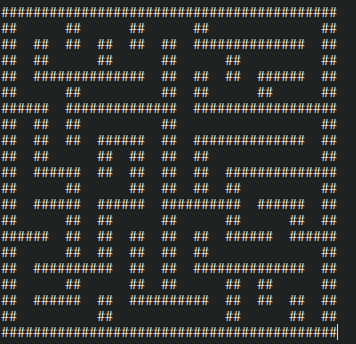# [Java] I tried to make a maze by the digging method ♪

This is the first post! !!

# I have a maze like this.# The reason I decided to make it

I used to make a maze game using the stick-down method with HTML5 and JS and exhibited it at a school festival. However, the maze created by the stick-down method has a very simple solution, so I wanted to try it with a digging method with a complicated solution.

# What is the digging method?

** ■ Very roughly speaking, the digging method is such an algorithm ** (Maybe it's wrong: sweat_drops :)

Create an odd-numbered map with a size of 5 or more both vertically and horizontally. Fill everything with a wall Randomly select places with odd X and Y coordinates other than the edges of the map and make holes. ★ Check if there are still walls with odd X and Y coordinates (this time the upper left corner is 0,0) other than the edge. ** If there is: ** Randomly select a place where the X and Y coordinates are both odd and already have holes, other than the edge of the map. | Check if there is still a wall 2 squares on all sides | ** If there is: ** Digging 2 squares at random from the direction of digging | If not **: Return to ** ★ ** If not: ** Maze completed

…… But it is difficult to implement it obediently, so I will devise various things.

# contents

## Main class

#### `MainClass.java`

``````
package automaze;
public class MainClass {
public static void main(String[] args) {
Maze maze=new Maze(21,21);//This time, I will make a 21x21 maze as a trial.
maze.show();
}
}
``````

## Maze class

#### `Maze.java`

``````
package automaze;

public class Maze {
private int pointX; //A mark for placing and erasing blocks.
private int pointY;
private int width; //Width and height.
private int height;
private byte[][] map; //Array to store the map
public Maze(int w, int h) { //Constructor
width = w;
height = h;
if (w % 2 != 0 && h % 2 != 0 && 5 <= w && 5 <= h) {
map = new byte[width][height];
make();
} else {
System.out.println("Create an odd number of 5 or more both vertically and horizontally.");
}
}

int randomPos(int muki) { //x,Returns odd random coordinates for both y coordinates
int result = 1 + 2 * (int) Math.floor((Math.random() * (muki - 1)) / 2);
return result;
}

private void make() { //Create a map

pointX = randomPos(width);
pointY = randomPos(height);

for (int y = 0; y < height; y++) { //Fill everything with a wall.
for (int x = 0; x < width; x++) {
map[x][y] = 1;
}
}
map[pointX][pointY] = 0;
dig();

}

private void dig() {
if (isAbleContinueDig() && map[pointX][pointY] == 0) {
map[pointX][pointY] = 0;
int direction = (int) Math.floor(Math.random() * 4);
switch (direction) {
case 0:
if (pointY != 1) {
if (map[pointX][pointY - 2] == 1) {
map[pointX][pointY - 1] = 0;
pointY -= 2;
break;//u
}
}
case 1:
if (pointY != height - 2) {
if (map[pointX][pointY + 2] == 1) {
map[pointX][pointY + 1] = 0;
pointY += 2;
break;//d
}
}
case 2:
if (pointX != 1) {
if (map[pointX - 2][pointY] == 1) {
map[pointX - 1][pointY] = 0;
pointX -= 2;
break;//l
}
}
case 3:
if (pointX != width - 2) {
if (map[pointX + 2][pointY] == 1) {
map[pointX + 1][pointY] = 0;
pointX += 2;
break;//r
}
}
}
map[pointX][pointY] = 0;
dig();
} else if (isAbleDig()) {
pointX = randomPos(width);
pointY = randomPos(height);
dig();
}

}

private boolean isAbleDig() { //See if there is still a place to dig
boolean result;
int cnt = 0;
for (int y = 0; y < height; y++) {
for (int x = 0; x < width; x++) {
if (x % 2 != 0 && y % 2 != 0) {

if (map[x][y] != 0) {
cnt++;
}
}
}
}
if (cnt == 0) {
result = false;
} else {
result = true;
}
return result;
}

private boolean isAbleContinueDig() {//Determine if there is any space left to dig in all directions

if (pointY != 1) {
if (map[pointX][pointY - 2] == 1) {
return true;
}
}
if (pointY != height - 2) {
if (map[pointX][pointY + 2] == 1) {
return true;
}
}
if (pointX != 1) {
if (map[pointX - 2][pointY] == 1) {
return true;
}
}
if (pointX != width - 2) {
if (map[pointX + 2][pointY] == 1) {
return true;
}
}
return false;
}

public void show() {
for (int y = 0; y < map.length; y++) {
System.out.println("");
for (int x = 0; x <map.length; x++) {

if (map[x][y] == 1) {
System.out.print("##");
} else {
System.out.print("  ");
}
}
}
}
public byte[][] getMaze() {
return map;
}

}
``````

# What I noticed when it was completed

I wonder if I can make it a little shorter. Also, maybe because I'm calling the digging function recursively, I get a stackoverflow error just by trying to create a slightly larger maze. I will edit this article as soon as I fix it. I am vaguely thinking that it seems quite so if I use a while statement.

# What you might do if you feel like it

--Start and goal are set automatically --Find the shortest path --Make it playable as a game.

# My environment

OS ：Windows10 IDE：Eclipse 2020-03